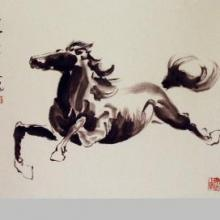# Less than ONE e- per ADU in a CMOS/CCD specs? does it make sense?

2 posts / 0 new
gblascoLess than ONE e- per ADU in a CMOS/CCD specs? does it make sense?

Hi,

I am thinking in buying a CMOS 14bit camera based in IMX178 MONO sensor.(ZWO ASI178M or QHY178M)

The specs for the QHYCCD Cold peltier model are here:

https://www.qhyccd.com/index.php?m=content&c=index&a=show&catid=94&id=42&cut=1

I will follow an example of a GAIN of 10, to the purpose of my question....

It is a 14bit camera, and you can read the SYSTEM GAIN at a graph in this image

It says that sensitivity is at GAIN 10: 0.28e- per ADU

https://www.qhyccd.com/index.php?m=content&c=index&a=show&catid=94&id=42&cut=1

I have checked also some specs for 16bits CCD cameras. there are many models with specs of 0.2-0.3 e- per ADU at a nominal GAIN

MY QUESTION:

Does it make sense have such much precision in the A/D converter at this gain? At first glampse the well at this GAIN is only about 3k e- , but ... Can we measure with a CMOS device less than 1e- ? Can we measure with a CCD device less than 1e-? Does it makes sense following the quantum mechanic principles?

It is a little mindblowing for me measure less than a electron or a photon. Does it make sense?

I have read about how to compute RMS noise, and we are near 1e- noise after summing with the Square root formula of several noises in this case. and I think is not very unlikely have about 1 to 1.5e- of noise.

With QE in IMX178 of about 80%. We can say that 10 photons are 8e-.... but if we have only 4 photons in the well? or does it make sense a difference between 1000 photons and 1004 photons?

AN EXAMPLE

If we have a pixel with a value in the 14bit converter of 20e- and another value in other pixel of 24e- with a RMS of 1.5e- , Are really a REAL difference between the two pixel measurements following the quantum mechanics rules, with a significant SIMGA value of confidence?

If not, it will be equivalent have a 12bits AD converter?

Why Am i doing this questions?

Does it really makes the difference a 14bit AD converter in this case or will we have the same confidence in the results with a 12bit AD converter?

This appy the same to the CCD 16bits sensors with a 0.2e- per ADU , does it make sense have a 16bit converter here, if we arent able to measure less than one photon/electron?

Thank you very much,

SFS
Since the fundamental

Since the fundamental physical process involves counting electrons, and electrons are elementary (i.e., cannot be subdivided into smaller components), there is no possibility of counting fractions of an electron.  When the sensitivity at some gain equates to less than one electron per ADU, what they are saying is that the gain setting is such that the detection of a single photon results in a multi-ADU signal.

There are a couple of facts that need being borne in mind.  First, the arrival of photons is a Poisson process, which entails fluctuations in arriving photons according to such a process, irrespective of other sources of variability such as atmospheric turbulence, detector and amplfier noise, and so on. Thus, one always wants to be in a situation where one is detecting large numbers of photons, so as to achieve a high SNR and consequently a small Cramér-Rao bound on the measurement variance. Second, the competent design of a signal processing system sets the least significant bit (LSB) so that it is randomly toggled by noise.  (More on the topic can be found on the Analog Devices Inc. web site.)  Increasing the gain to toggle a higher order bit results in a reduction in the spur free dynamic range, but can be partially compensated for by increasing the number of bits of the ADC.

Additionally, having more ADC bits increases the linear dynamic range (for a given gain setting) and reduces the impact of quantization noise.  There is no reason whatsoever, aside possibly from cost, for choosing a 12 bit ADC over a 14 bit ADC of equal quality.  More bits are better!

Hope that answered your question!  Do check out the tutorials at https://www.analog.com/en/index.html